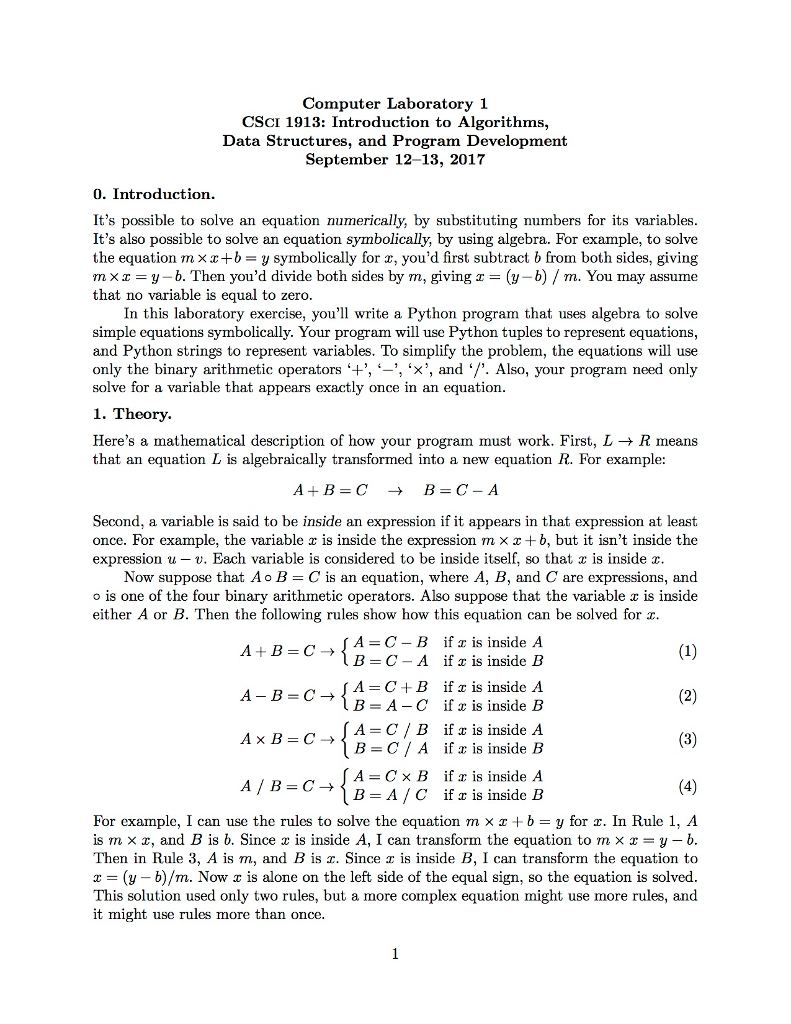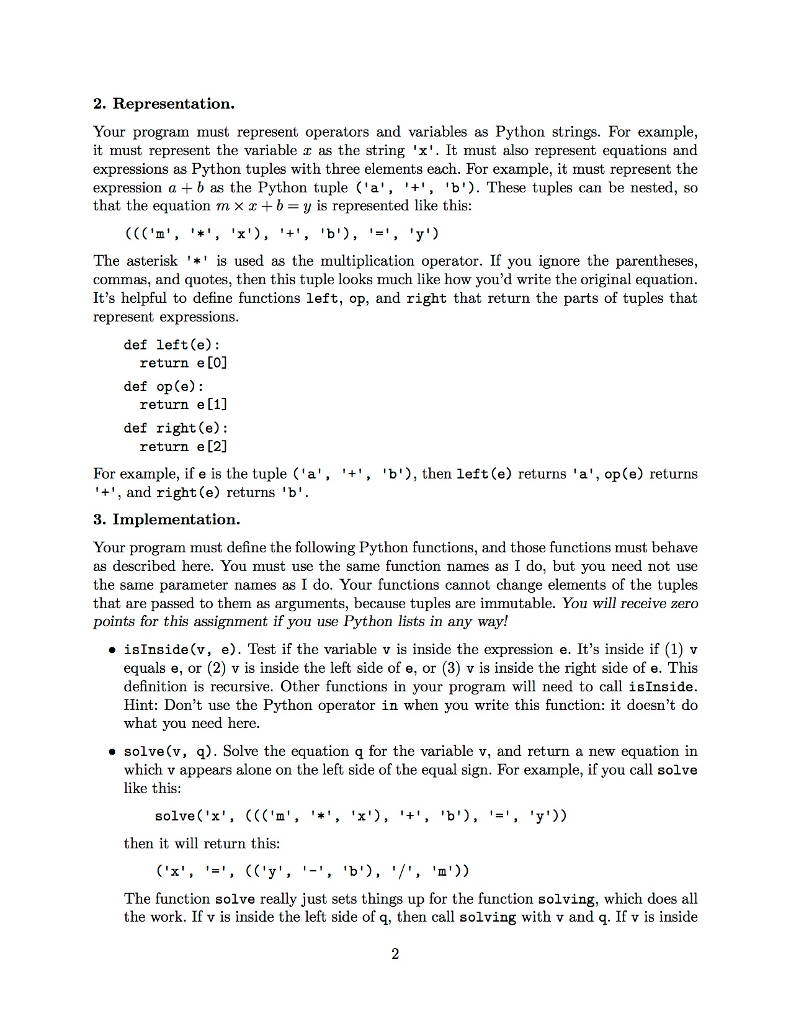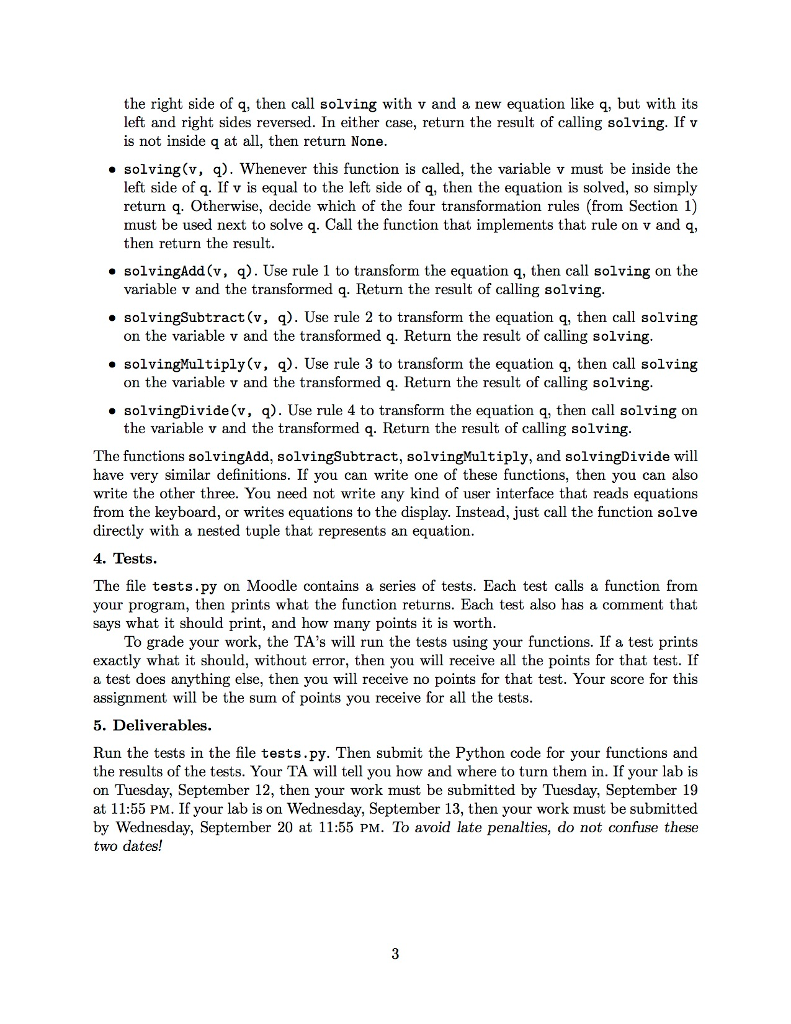# (Solved Homework): Computer Laboratory 1 CSCI 1913: Introduction to Algorithms, Data Structures, and Program Development September 12-13, 2017 0. Introductio…Computer Laboratory 1 CSCI 1913: Introduction to Algorithms, Data Structures, and Program Development September 12-13, 2017 0. Introduction. It’s possible to solve an equation numerically, by substituting numbers for its variables It’s also possible to solve an equation symbolically, by using algebra. For example, to solve the equation m ×x+b y symbolically for x, you’d first subtract b from both sides, giving m × z = y-b. Then you’d divide both sides by m, giving z (y-b) / m. You may assume that no variable is equal to zero. In this laboratory exercise, you’ll write a Python program that uses algebra to solve simple equations symbolically. Your program will use Python tuples to represent equations, and Python strings to represent variables. To simplify the problem, the equations will use only the binary arithmetic operators 4, , ‘x’, andゾ. Also, your program need only solve for a variable that appears exactly once in an equation. 1. Theory Here’s a mathematical description of how your program must work. First, L → R means that an equation L is algebraically transformed into a new equation R. For example: Second, a variable is said to be inside an expression if it appears in that expression at least once. For example, the variable x is inside the expression m × x + b, but it isn’t inside the expression u – v. Each variable is considered to be inside itself, so that x is inside x Now suppose that Ao B = C is an equation, where A, B, and C are expressions, and suppose that the variable a is inside o is one of the four binary arithmetic operators. Also either A or B. Then the following rules show how this equation can be solved for x A = C-B B if z is inside A is inside B /1 + B = C → C-A if A-B-C^[A-C+B if z is inside A B-A-C A C / B B = C / A A=C× B if z is inside B if is inside A if x is inside B ifx is inside A A/B=C→ B=A/C ifxīs inside B For example, I can use the rules to solve the equation m × z + b = y for z. In Rule 1, A is m × x, and B is b. Since is inside A, I can transform the equation to m × = y-b Then in Rule 3, A is m, and B is z. Since r is inside B, I can transform the equation to x -(y -b)/m. Now x is alone on the left side of the equal sign, so the equation is solved This solution used only two rules, but a more complex equation might use more rules, and it might use rules more than once.

Don't use plagiarized sources. Get Your Custom Essay on
(Solved Homework): Computer Laboratory 1 CSCI 1913: Introduction to Algorithms, Data Structures, and Program Development September 12-13, 2017 0. Introductio…
Get an essay WRITTEN FOR YOU, Plagiarism free, and by an EXPERT!

def left(e):
return e
def right(e):
return e
def op(e):
return e

def isInside(v,e):
if type(e) != tuple:
if v == e:
return True
else:
return False
else:
if isInside(v,left(e)):
return True
if isInside(v,right(e)):
return True
return False

def solve(v,q):
if isInside(v,left(q)):
return solving(v,q)
elif isInside(v,right(q)):
r = (right(q),op(q),left(q))
return solving(v,r)
else:
return None

def solving(v,q):
if v == left(q):
return q
elif op(left(q)) == ‘+’:
elif op(left(q)) == ‘-‘:
return solvingSubtract(v,q)
elif op(left(q)) == ‘*’:
return solvingMultiply(v,q)
elif op(left(q)) == ‘/’:
return solvingDivide(v,q)

if isInside(v,left(left(q))):
return (left(left(q)), ‘=’, (right(q), ‘-‘,right(left(q))))
elif isInside(v,right(left(q))):
return (right(left(q)), ‘=’,(right(q), ‘-‘,left(left(q))))
def solvingSubtract(v,q):
if isInside(v,left(left(q))):
return (left(left(q)),’=’,(right(q), ‘+’,right(left(q))))
elif isInside(v,right(left(q))):
return (right(left(q)),’=’,(right(left(q)),’-‘, right(q)))
def solvingMultiply(v,q):
if isInside(v,left(left(q))):
return (left(left(q)), ‘=’, (right(q), ‘/’,right(left(q))))
elif isInside(v,right(left(q))):
return (right(left(q)), ‘=’,(right(q), ‘/’,left(left(q))))
def solvingDivide(v,q):
if isInside(v,left(left(q))):
return (left(left(q)),’=’,(right(q), ‘*’,right(left(q))))
elif isInside(v,right(left(q))):
return (right(left(q)),’=’,(right(left(q)),’/’, right(q)))

print(isInside(‘x’, ‘x’))                          # True
print(isInside(‘x’, ‘y’))                          # False
print(isInside(‘x’, (‘x’, ‘+’, ‘y’)))              # True
print(isInside(‘x’, (‘a’, ‘+’, ‘b’)))              # False
print(isInside(‘x’, ((‘m’, ‘*’, ‘x’), ‘+’, ‘b’))) # True

print(solve(‘x’, ((‘a’, ‘+’, ‘x’), ‘=’, ‘c’))) # (‘x’, ‘=’, (‘c’, ‘-‘, ‘a’))
print(solve(‘x’, ((‘x’, ‘+’, ‘b’), ‘=’, ‘c’))) # (‘x’, ‘=’, (‘c’, ‘-‘, ‘b’))

print(solve(‘x’, ((‘a’, ‘-‘, ‘x’), ‘=’, ‘c’))) # (‘x’, ‘=’, (‘a’, ‘-‘, ‘c’))
print(solve(‘x’, ((‘x’, ‘-‘, ‘b’), ‘=’, ‘c’))) # (‘x’, ‘=’, (‘c’, ‘+’, ‘b’))

print(solve(‘x’, ((‘a’, ‘*’, ‘x’), ‘=’, ‘c’))) # (‘x’, ‘=’, (‘c’, ‘/’, ‘a’))
print(solve(‘x’, ((‘x’, ‘*’, ‘b’), ‘=’, ‘c’))) # (‘x’, ‘=’, (‘c’, ‘/’, ‘b’))

print(solve(‘x’, ((‘a’, ‘/’, ‘x’), ‘=’, ‘c’))) # (‘x’, ‘=’, (‘a’, ‘/’, ‘c’))
print(solve(‘x’, ((‘x’, ‘/’, ‘b’), ‘=’, ‘c’))) # (‘x’, ‘=’, (‘c’, ‘*’, ‘b’))

print(solve(‘x’, (‘y’, ‘=’, ((‘m’, ‘*’, ‘x’), ‘+’, ‘b’))))
# (‘x’, ‘=’, ((‘y’, ‘-‘, ‘b’), ‘/’, ‘m’)Pages (550 words)
Approximate price: -

Why Work with UsTop Quality and Well-Researched Papers

We always make sure that writers follow all your instructions precisely. You can choose your academic level: high school, college/university or professional, and we will assign a writer who has a respective degree.We have a team of professional writers with experience in academic and business writing. Many are native speakers and able to perform any task for which you need help.Free Unlimited Revisions

If you think we missed something, send your order for a free revision. You have 10 days to submit the order for review after you have received the final document. You can do this yourself after logging into your personal account or by contacting our support.Prompt Delivery and 100% Money-Back-Guarantee

All papers are always delivered on time. In case we need more time to master your paper, we may contact you regarding the deadline extension. In case you cannot provide us with more time, a 100% refund is guaranteed.Original & Confidential

We use several writing tools checks to ensure that all documents you receive are free from plagiarism. Our editors carefully review all quotations in the text. We also promise maximum confidentiality in all of our services.Our support agents are available 24 hours a day 7 days a week and committed to providing you with the best customer experience. Get in touch whenever you need any assistance.

Try it now!

## Calculate the price of your order

Total price:
\$0.00

How it works?Fill in the order form and provide all details of your assignment.Proceed with the payment

Choose the payment system that suits you most.Our Services

No need to work on your paper at night. Sleep tight, we will cover your back. We offer all kinds of writing services.## Essay Writing Service

No matter what kind of academic paper you need and how urgent you need it, you are welcome to choose your academic level and the type of your paper at an affordable price. We take care of all your paper needs and give a 24/7 customer care support system.An admission essay is an essay or other written statement by a candidate, often a potential student enrolling in a college, university, or graduate school. You can be rest assurred that through our service we will write the best admission essay for you.Editing Support

Our academic writers and editors make the necessary changes to your paper so that it is polished. We also format your document by correctly quoting the sources and creating reference lists in the formats APA, Harvard, MLA, Chicago / Turabian.Revision Support

If you think your paper could be improved, you can request a review. In this case, your paper will be checked by the writer or assigned to an editor. You can use this option as many times as you see fit. This is free because we want you to be completely satisfied with the service offered.